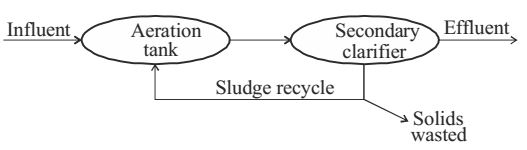## Municipal solid waste miscellaneous

#### Municipal Solid Waste

1. 45. A landfill is to be designed to serve a population of 200000 for a period of 25 years. The solid waste (SW) generation is 2 kg/person/day. The density of the uncompacted SW is 100 kg/m³ and a compaction ratio of 4 is suggested. The ratio of compacted fill (i.e, SW+ cover) to compacted SW is 1.5. The landfill volume (in million m³) required is __________.

1. 21.9 Total waste Population = 2 × 105
Waste generation/day = 2kg × 2 × 105
= 4 × 105kg/ day
For 25 years,
Total waste = 4 × 105 × 25 × 365
= 365 × 107 kg/25 years

 Compaction ratio = Vafter compaction = V' = 0.4 Vbefore compaction V

 V = 365 × 107 = 365 × 105 m³ 100

V' = 0.4 × 365 × 105 = 1.46 × 105
 sw + cover = sw + cover = 1.5 sw sw sw

 ∴ cover = 0.5 sw

∴ Cover = 0.5 × sw = 0.5 × 146 × 105
= 73 × 105
∴ Total volume = (146 + 73) × 105
= 219 × 105
= 21.9 × 106
= 21.9 million m³

##### Correct Option: C

21.9 Total waste Population = 2 × 105
Waste generation/day = 2kg × 2 × 105
= 4 × 105kg/ day
For 25 years,
Total waste = 4 × 105 × 25 × 365
= 365 × 107 kg/25 years

 Compaction ratio = Vafter compaction = V' = 0.4 Vbefore compaction V

 V = 365 × 107 = 365 × 105 m³ 100

V' = 0.4 × 365 × 105 = 1.46 × 105
 sw + cover = sw + cover = 1.5 sw sw sw

 ∴ cover = 0.5 sw

∴ Cover = 0.5 × sw = 0.5 × 146 × 105
= 73 × 105
∴ Total volume = (146 + 73) × 105
= 219 × 105
= 21.9 × 106
= 21.9 million m³

Direction: An activated sludge system (sketched below) is operating at equilibrium with the following information. Waste water related data: flow rate = 500 m³/hour, influent BOD = 150 mg/l, effluent BOD = 10 mg/l. Aeration tank related data: hydraulic retention time = 8 hours, mean-cell-residence time = 240 hours, volume = 4000 m³, mixed liquor suspended solids = 2000 mg/l.1. The mass (in kg/day) of solids wasted from the system is

1.  Sludge age (Qc) = Mass of biomass in system Mass of biomass leaving the system

Therefore,
 Mass of biomass leaving system = Mass of biomass in system Qc

 = Volume × MLSS Qc

 = 4000 × 10-3 2000 × 10-6 240

 = 8000 kg/l = 800 kg/day. 240

##### Correct Option: C

 Sludge age (Qc) = Mass of biomass in system Mass of biomass leaving the system

Therefore,
 Mass of biomass leaving system = Mass of biomass in system Qc

 = Volume × MLSS Qc

 = 4000 × 10-3 2000 × 10-6 240

 = 8000 kg/l = 800 kg/day. 240

1. The food-to-biomass (F/M) ratio (in kg BOD per kg biomass per day) for the aeration tank is

1.  F = Q × BOD m V × MLSS

 = 500 × 150 4000 × 200

= 9.375 × 10–3 per hour
= 0.225 per day.

##### Correct Option: C

 F = Q × BOD m V × MLSS

 = 500 × 150 4000 × 200

= 9.375 × 10–3 per hour
= 0.225 per day.

Direction: The sludge from the aeration tank of the activated sludge process (ASP) has solids content (by weight) of 2%. This sludge is put in a sludge thickener, where sludge volume is reduced to half. Assume that the amount of solids in the supernatant from the thickener is negligible, the specific gravity of sludge solids is 2.2 and the density of water is 1000 kg/m³.

1. What is the solids content (by weight) of the thickened sludge?

1.  Solid content = Weight of solid Total weight

 = 0.02 × V × ρ1Vρ1 - V ρw2

 = 0.02 × V × ρ1 ≈ 3.96 % (1011.03 - 500)

##### Correct Option: A

 Solid content = Weight of solid Total weight

 = 0.02 × V × ρ1Vρ1 - V ρw2

 = 0.02 × V × ρ1 ≈ 3.96 % (1011.03 - 500)

1. What is the density of the sludge removed from the aeration tank?

1.  Density = Total weight Volume sludge water

 = W Vsludge + Vwater

 = W .02w + .98w GPw Pw

= 1011.03 kg/m³.

##### Correct Option: C

 Density = Total weight Volume sludge water

 = W Vsludge + Vwater

 = W .02w + .98w GPw Pw

= 1011.03 kg/m³.